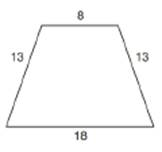Chapter 8.5, Problem 27EElementary Geometry For College St...

7th Edition
Alexander + 2 others
ISBN: 9781337614085

Solutions

Chapter
SectionElementary Geometry For College St...

7th Edition
Alexander + 2 others
ISBN: 9781337614085
Textbook Problem

A circle can be inscribed in the trapezoid shown. Find the area of that circle.To determine

To find:

Area of circle inscribed in a trapezoid.

Explanation

Formula:

If r is the radius of circle,

Acircle=πr2.

Calculation:

Consider the following diagram in which ABCD is a trapezoid with an inscribed circle.

Two perpendiculars AM and DN have been drawn from vertices A and D of the trapezoid.

Consider AMB and DNC.

In these two triangles, AB=DC=13

AMB=DNC=90°.

We know that the distance between parallel lines is same.

Hence, AM=DN.

From the above observations, it is clear that AMBDNC by RHS (right-angle hypotenuse side) test of congruency.

We know that, if two triangles are congruent then their corresponding sides are also equal.

Hence, BM=NC.

In quadrilateral AMND, AM=DN and AMN=DNM=90°,

Hence, AMND is a rectangle.

Opposite sides of a rectangle are equal. Therefore, AD=MN=8

Points M and N lie on BC. Hence, BC can be written as the sum of three segments.

BC=BM+MN+NC

18=BM+8+NC

Thus, BM+NC=18-8=10

Since, BM=NC

Still sussing out bartleby?

Check out a sample textbook solution.

See a sample solution

The Solution to Your Study Problems

Bartleby provides explanations to thousands of textbook problems written by our experts, many with advanced degrees!

Get Started

Find more solutions based on key concepts